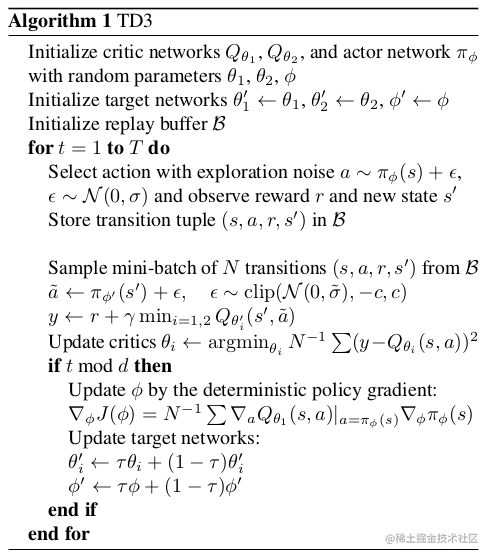# 强化学习 14 —— TD3 算法详解与tensorflow 2.0 实现

TD3算法主要对DDPG做了三点改进，将会在下面 一一讲解，两者的代码也很相似，这里只展示改进的部分，如果对DDPG算法不太熟悉可以参考上一篇博客强化学习 13——DDPG算法详解与实战

1、双 Critic 网络

$E_\epsilon[max_{a'}(Q(s',a')+\epsilon)] \geq max_{a'}Q(s',a')$

self.q_net1 = QNetwork(state_dim, action_dim, hidden_dim)
self.q_net2 = QNetwork(state_dim, action_dim, hidden_dim)
self.target_q_net1 = QNetwork(state_dim, action_dim, hidden_dim)
self.target_q_net2 = QNetwork(state_dim, action_dim, hidden_dim)
self.policy_net = PolicyNetwork(state_dim, action_dim, hidden_dim, action_range)
self.target_policy_net = PolicyNetwork(state_dim, action_dim, hidden_dim, action_range)

target_q_min = tf.minimum(self.target_q_net1(target_q_input), 		               self.target_q_net2(target_q_input))
target_q_value = reward + (1 - done) * gamma * target_q_min

2、延迟 Actor 网络更新

TD3中使用的第二个技巧就是对Policy进行延时更新。在双网络中，我们让target网络与当前网络更新不同步，当前网络更新 d 次之后在对target网络进行更新（复制参数）。这样就可以减少积累误差，从而降低方差。同样的我们也可以policy网络进行延时更新，因为actor-critic方法中参数更新缓慢，进行延时更新一方面可以减少不必要的重复更新，另一方面也可以减少在多次更新中累积的误差。在降低更新频率的同时，还应使用软更新：

$\theta'\leftarrow \tau\theta + (1-\tau)\theta'$

if self.update_cnt % self.policy_target_update_interval == 0

3、目标策略的平滑正则化 Target Policy Smoothing Regularization

$y = r + \gamma\;Q_{\theta'}(s', \pi_{\phi'}(s')+\epsilon)\;\; \epsilon ～clip(N(0,\sigma), -c, c)$

def evaluate(self, state, eval_noise_scale):
state = state.astype(np.float32)
action = self.forward(state)
action = self.action_range * action
normal = Normal(0, 1)
noise = normal.sample(action.shape) * eval_noise_scale
eval_noise_clip = 2 * eval_noise_scale
noise = tf.clip_by_value(noise, -eval_noise_clip, eval_noise_clip)
action = action + noise
return actionzhuanlan.zhihu.com/p/55307499

zhuanlan.zhihu.com/p/86297106?…